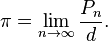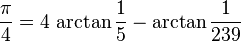## Calculating PiSo, I was writing some program and I needed π for something – I’m being vague because truth be told, I can’t remember what I was originally trying to do. We all know π to be 3.1315… but again, for some obscure reason, I needed a little more precision. Now, I know I could have simply gone and used 22/7, or looked it up – there are many resources on the web which will give you π to any precision you desire, but how do you calculate it? Given the news of Wolfram Alpha’s launch, and its tendency towards math, I thought I’d ask it how to Calculate π.So, what does this really mean? There’s a great write up of this theory on Knott’s page, and of course on our old friend Wikipedia, but simply the logic is that the ratio between the circumference and radius of a circle (π) can be bound by the perimeters of two polygons, one inscribed outside the circle, one inside:Simple pentagon approximation of Pi

you can see that as the polygons gain sides, the area between them shrinks, thus the approximation of π gets more accurate (remember, you can accurately calculate the perimeter of a polygon simply by multiplying the number of sides by the length of each). in 480AD, a Chinese mathmatician Zu Chongzhi prooved that 223/71 < π < 22/7 by using 96 side polygons. This is 3.1419 – pretty good for the time.

You can read more on Wikipedia so I won’t bore you here, only to say that rather than using polygons, you can use trigonometry to do the same thing – One of the most understandable algorithms was desribed by John Machin in 1706:And this is the one I decided to use.

You can download a copy of the VBScript class I finally ended up with from my Google site – it will calcuate π to any number of places you want.

Now, if I could only remember what I needed it for in the first place…